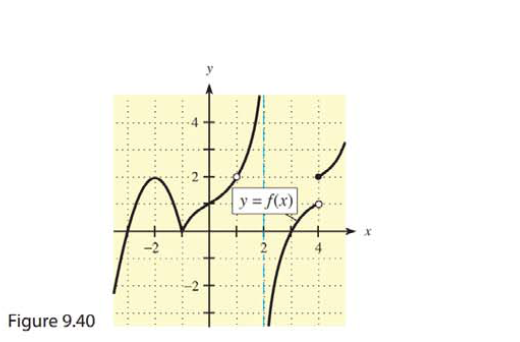Chapter 9, Problem 5RE### Mathematical Applications for the ...

11th Edition
Ronald J. Harshbarger + 1 other
ISBN: 9781305108042

#### Solutions

Chapter
Section### Mathematical Applications for the ...

11th Edition
Ronald J. Harshbarger + 1 other
ISBN: 9781305108042
Textbook Problem

# In Problems 1-6, use the graph of y = f ( x ) in Figure 9.40 to find the functional values and limits, if they exist.(a) f ( 1 ) (b) lim x → 1 f ( x )

(a)

To determine

The value of f(1) by using the provided graph.

Explanation

Given Information:

The graph of y=f(x) is shown below,

The function is f(1).

Explanation:

Consider the provided graph,

(b)

To determine

The value of limx1f(x) by using the provided graph.

### Still sussing out bartleby?

Check out a sample textbook solution.

See a sample solution

#### The Solution to Your Study Problems

Bartleby provides explanations to thousands of textbook problems written by our experts, many with advanced degrees!

Get Started